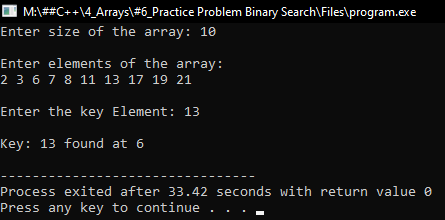# Binary Search in C++

## Binary Search in C++ with Examples:

In this article, I am going to discuss the Binary Search in C++ Language with examples. Please read our previous articles, where we discussed Linear Search in C++ Language with examples.

##### Binary Search in C++:

Here, we will discuss Binary Search. Already we have learned about the linear search in the previous article. Now, this is another method of searching. Now in this search method, one compulsory condition that is the elements must be in sorted order. Then only you can perform the binary search.

##### What is Binary Search?

Suppose you have a book if you want to search for any page number, let us suppose we want to go on page number 125. So how will you search? Do you search like this 1, 2, and so on? No. If you’re going like this then this is a linear search.

But how do we search for page number 125 in a book? We simply open the book from something like 200, so 200 means 125 is on the left-hand side. So, we should search on the left-hand side. Let us say we are opening the book again like middle right now and the page number is 67. So, 125 will be on the right-hand side.

How do we know that is on the left-hand side or right turn side? Because these page numbers are sorted. This is the method that we follow because the page numbers are sorted the same idea is used by binary search. So let us learn binary search through an example.

##### How does Binary Search work?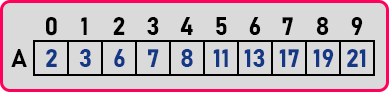Here is the list of elements and we have to search for a key ‘13’. Now I need two things for searching that is starting element and the ending element that is low and high. So initially low is 0th and high is on 9th index,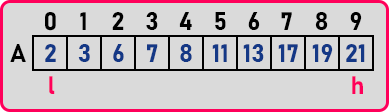Then, we have to search in the middle. We have to find one more thing that is middle, that middle is ‘(low + high) / 2’. And that is float value means we have to truncate the decimal point if I get any decimal point.

###### Step1:

So first of all, we have the search for 13 so find out mid as,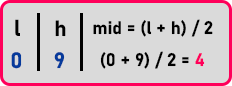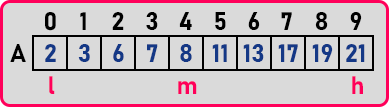mid = 4, is mid pointing the key element? No, we are searching for ‘13’ and mid is pointing on ‘8’. Now where we should search. We should search on the right-hand side. Now where low will start now? Low should at after mid, don’t include mid because already we have checked it. So low will be from 5th (mid + 1) index and high will be remains same on 9th index.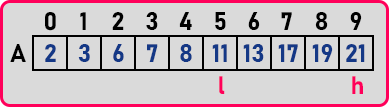###### Step2:

Now again we will find mid as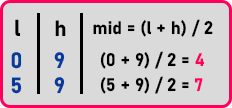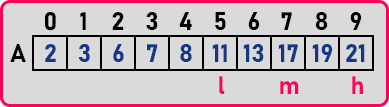mid = 7, is mid pointing the key element? No, we are searching for ‘13’ and mid is pointing on ‘17’. 13 < 17, so search on the left-hand side. So, what do you have to change now? Low will remain unchanged and high should be changed to mid-1.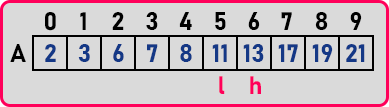###### Step3:

Now again we will find mid as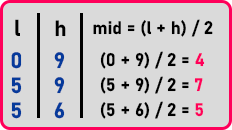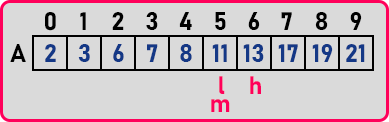Mid is ‘(5 + 6) / 2 = 5.5’ but we will truncate decimal and take it as 5. So, we got mid as same as low. Now check at the mid, is it the element that we are searching for that is 11 and we are searching for 13.

Our element is on the right-hand side so check on the right-hand side in the list. So, what should we modify? Low should be changed to mid + 1, that is 6. L = 6 and h = 6. So, both are in the same place. So mid is also 6.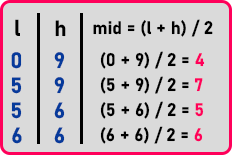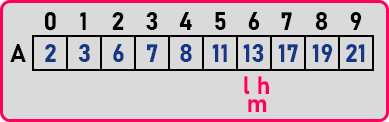Is it the element that you are searching for? Yes, it is ‘13’. We got the key. In how many comparisons do we get the key? We perform 4 comparisons. If we perform a linear search then we’ll be checking all these. Binary search is faster. So, this is how binary works.

Now one more thing is an unsuccessful search. In Binary Search if low became greater than high means Element is not there. This will be an unsuccessful search. This is a procedure of binary search. Now let us write a program and show you in the program how to calculate mid every time and update low and high.

##### Program for Binary Search in C++:
```#include <iostream>
#include <conio.h>
using namespace std;
int main()
{
int n, l, mid, h, key;
cout << "Enter size of the array: ";
cin >> n;
cout << endl;
int A[n];
cout <<"Enter elements of the array:\n";
for (int i = 0; i < n; i++)
{
cin >> A[i];
}

cout <<"\nEnter the key Element: ";
cin >> key;
cout << endl;

l = 0;
h = n - 1;

while(l <= h)
{
mid = (l + h) / 2;
if(key == A[mid])
{
cout << "Key: " << key << " found at " << mid << endl;
return 0;
}
else if (key < A[mid])
h = mid - 1;
else
l = mid + 1;
}